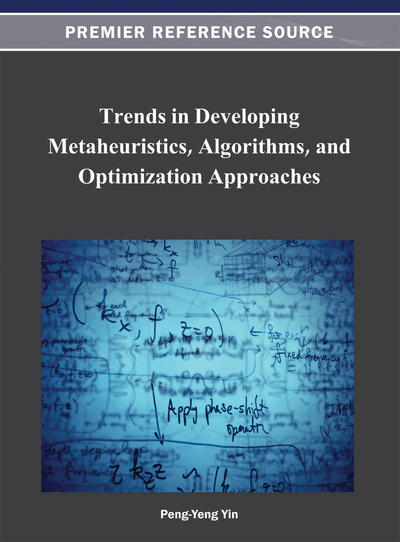# A Novel Particle Swarm Optimization Algorithm for Multi-Objective Combinatorial Optimization Problem

Rahul Roy (KIIT University, India), Satchidananda Dehuri (Fakir Mohan University, India) and Sung Bae Cho (Yonsei University, South Korea)
DOI: 10.4018/978-1-4666-2145-9.ch001
Available
\$37.50
No Current Special Offers

## Abstract

The Combinatorial problems are real world decision making problem with discrete and disjunctive choices. When these decision making problems involve more than one conflicting objective and constraint, it turns the polynomial time problem into NP-hard. Thus, the straight forward approaches to solve multi-objective problems would not give an optimal solution. In such case evolutionary based meta-heuristic approaches are found suitable. In this paper, a novel particle swarm optimization based meta-heuristic algorithm is presented to solve multi-objective combinatorial optimization problems. Here a mapping method is considered to convert the binary and discrete values (solution encoded as particles) to a continuous domain and update it using the velocity and position update equation of particle swarm optimization to find new set of solutions in continuous domain and demap it to discrete values. The performance of the algorithm is compared with other evolutionary strategy like SPEA and NSGA-II on pseudo-Boolean discrete problems and multi-objective 0/1 knapsack problem. The experimental results confirmed the better performance of combinatorial particle swarm optimization algorithm.
Chapter Preview
Top

## Introduction

Many real world decision making problems involve discrete and disjunctive choices, i.e., such problems’ solution can be either represented by 0/1 (represented as yes/ no) or an integer number. These problems are known as combinatorial problems. When these problems are modelled with more than one conflicting objectives and constraints, which need to be satisfied for finding optimal solutions, such problems are called multi-objective combinatorial optimization (MOCO) problems. Garey and Johnson (1990) showed that the decision making problems are intractable in nature. In case of such problems, the use of exact methods that guarantee generation of exact Pareto-optimal solutions may be not possible because of computational requirements of the methods. To solve such problems, many meta-heuristic algorithms have been found in the specialized literature (Zitzler & Thiele, 1998; Deb, Pratap, Agrawal, & Meyarivan, 2002; Jaszkiewicz, 2000). These algorithms aim at effective generation of subset of Pareto-optimal solutions which is an approximate representation of the whole Pareto set. Laumanns et al. (2002) compared the performance of multi-objective evolutionary algorithms on the pseudo-Boolean functions. In this comparative study, they analyzed the running time of the evolutionary based algorithms. Jaszkiewicz (2000) proposed multi-objective genetic local search algorithm and evaluated the performance with the multi-objective 0/1 knapsack problem. Zitzler et al. (1999) implemented some of the state-of-the-art combinatorial optimization problems with multi-objective genetic algorithm. Later Grosan et al. (2003) proposed a multi-objective evolutionary algorithm based on Є-relation for solving multi-objective 0/1 knapsack problem.

In recent days, multi-objective particle swarm optimization (MOPSO) based meta-heuristics algorithm have attained a great attention among the researchers. After the pioneering work by Coello and Lechuga (2002), it has been used in various domain to solve multi-objective problems. But the algorithm is designed to solve real valued problems. However, the algorithm can be used to solve combinatorial problems by truncating the real valued solution to integer. But it would incur a truncation error and will create a hindrance in convergence of the solutions to the true Pareto front.

Thus, an attempt has been made by the authors to solve a combinatorial optimization problems by designing a binary MOPSO (BMOPSO)(Das, Roy, Dehuri, & Cho, 2011).

This algorithm works well for problems where the solution can be represented as strings of 0/1. However, the solutions which are represented as integers, this approach would need to convert the integer values to binary form before they are used for updation. This would incur an updation cost overhead based on number of bits required to represent the solution in each dimension. This motivated us to design a combinatorial MOPSO (CMOPSO) algorithm that can overcome both drawbacks stated above for using real valued or binary MOPSO for solving combinatorial optimization problem whose solution can be represented as integers. During the study of different MOPSO algorithms, we have seen that only the position updation equation, which generates the new solutions, depends on the solutions space. However, all other components, like the gbest selection, repository updation, etc., depend on the objective space which is real valued. Thus we can conclude from this observation that to design a combinatorial optimization algorithm, we need to concentrate more on position update equation whereas the rest of the strategies presented in real valued MOPSO literature can be adapted directly in the algorithm. This motivated us to present a new position update strategy that can adapt to the integer valued solution space without truncating of transforming to binary form for solving the integer valued combinatorial optimization problems. An empirical comparison of the algorithm with binary MOPSO, NSGA-II (Deb et al., 2002) and SPEA (Zitzler & Thiele, 1998) is done to evaluate the performance on the pseudo-Boolean function and multi-objective 0/1 knapsack problem.

## Complete Chapter List

Search this Book:
Reset